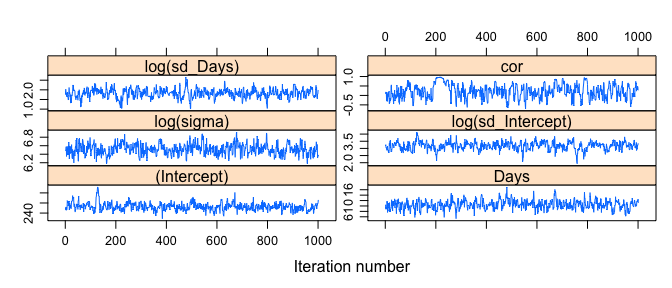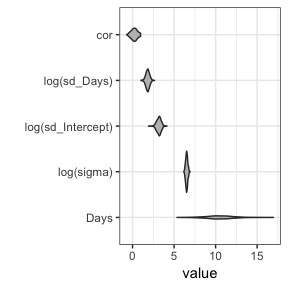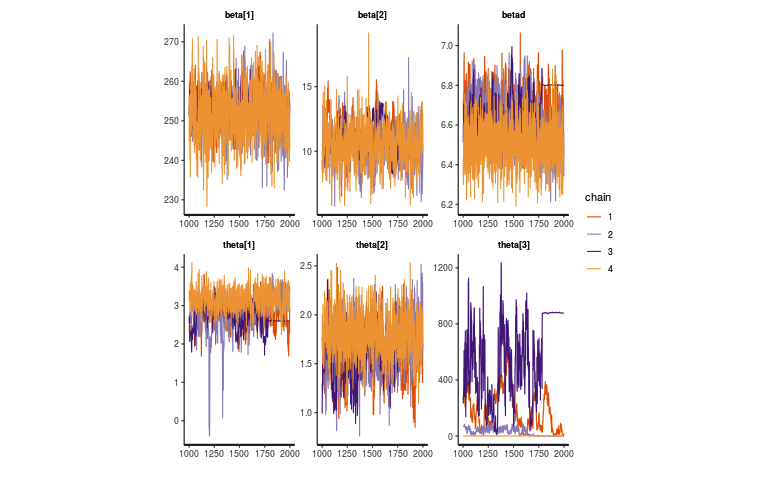# post-hoc MCMC with glmmTMB

#### 2019-01-11

One commonly requested feature is to be able to run a post hoc Markov chain Monte Carlo analysis based on the results of a frequentist fit. This is often a reasonable shortcut for computing confidence intervals and p-values that allow for finite-sized samples rather than relying on asymptotic sampling distributions. This vignette shows an example of such an analysis. Some caveats:

• when using such a “pseudo-Bayesian” approach, be aware that using a scaled likelihood (implicit, improper priors) can often cause problems, especially when the model is poorly constrained by the data
• in particular, models with poorly constrained random effects (singular or nearly singular) are likely to give bad results
• as shown below, even models that are well-behaved for frequentist fitting may need stronger priors to give well-behaved MCMC results
• as with all MCMC analysis, it is the user’s responsibility to check for proper mixing and convergence of the chains before drawing conclusions
• the first MCMC sampler illustrated below is simple (Metropolis with a multivariate normal candidate distribution). Users who want to do MCMC sampling on a regular basis should consider the tmbstan package, which does much more efficient hybrid/Hamiltonian Monte Carlo sampling.

library(glmmTMB)
library(coda)     ## MCMC utilities
library(reshape2) ## for melt()
## graphics
library(lattice)
library(ggplot2); theme_set(theme_bw())

Fit basic model:

data("sleepstudy",package="lme4")
fm1 <- glmmTMB(Reaction ~ Days + (Days|Subject),
sleepstudy)

Set up for MCMC: define scaled log-posterior function (in this case the log-likelihood function); extract coefficients and variance-covariance matrices as starting points.

## FIXME: is there a better way for user to extract full coefs?
rawcoef <- with(fm1$obj$env,last.par[-random])
names(rawcoef) <- make.names(names(rawcoef),unique=TRUE)
## log-likelihood function
## (MCMCmetrop1R wants *positive* log-lik)
logpost_fun <- function(x) -fm1$obj$fn(x)
## check definitions
stopifnot(all.equal(c(logpost_fun(rawcoef)),
c(logLik(fm1)),
tolerance=1e-7))
V <- vcov(fm1,full=TRUE)

This is a basic block Metropolis sampler, based on Florian Hartig’s code here.

##' @param start starting value
##' @param V variance-covariance matrix of MVN candidate distribution
##' @param iterations total iterations
##' @param nsamp number of samples to store
##' @param burnin number of initial samples to discard
##' @param thin thinning interval
##' @param tune tuning parameters; expand/contract V
##' @param seed random-number seed
run_MCMC <- function(start,
V,
logpost_fun,
iterations = 10000,
nsamp = 1000,
burnin = iterations/2,
thin = floor((iterations-burnin)/nsamp),
tune = NULL,
seed=NULL
) {
## initialize
if (!is.null(seed)) set.seed(seed)
if (!is.null(tune)) {
tunesq <- if (length(tune)==1) tune^2 else outer(tune,tune)
V <-  V*tunesq
}
chain <- matrix(NA, nsamp+1, length(start))
chain[1,] <- cur_par <- start
postval <- logpost_fun(cur_par)
j <- 1
for (i in 1:iterations) {
proposal = MASS::mvrnorm(1,mu=cur_par, Sigma=V)
newpostval <- logpost_fun(proposal)
accept_prob <- exp(newpostval - postval)
if (runif(1) < accept_prob) {
cur_par <- proposal
postval <- newpostval
}
if ((i>burnin) && (i %% thin == 1)) {
chain[j+1,] <- cur_par
j <- j + 1
}
}
chain <- na.omit(chain)
colnames(chain) <- names(start)
chain <- coda::mcmc(chain)
return(chain)
}

Run the chain:

t1 <- system.time(m1 <- run_MCMC(start=rawcoef,
V=V, logpost_fun=logpost_fun,
seed=1001))

(running this chain takes 13.2 seconds)

colnames(m1) <- c(names(fixef(fm1)[]),
"log(sigma)",
c("log(sd_Intercept)","log(sd_Days)","cor"))
m1[,"cor"] <- sapply(m1[,"cor"],get_cor)
xyplot(m1,layout=c(2,3),asp="fill")The trace plots are poor, especially for the correlation; the effective sample size backs this up, as would any other diagnostics we did.

print(effectiveSize(m1),digits=3)
##       (Intercept)              Days        log(sigma) log(sd_Intercept)
##               142               227               208               152
##      log(sd_Days)               cor
##               170               107

In a real analysis we would stop and fix the mixing/convergence problems before proceeding; for this simple sampler, some of our choices would be (1) simply run the chain for longer; (2) tune the candidate distribution (e.g. by using tune to scale some parameters, or perhaps by switching to a multivariate Student t distribution [see the mvtnorm package]); (3) add regularizing priors.

Ignoring the problems and proceeding, we can compute column-wise quantiles or highest posterior density intervals (coda::HPDinterval) to get confidence intervals. Plotting posterior distributions, omitting the intercept because it’s on a very different scale.## tmbstan

The tmbstan package allows direct, simple access to a hybrid/Hamiltonian Monte Carlo algorithm for sampling from a TMB object; the $obj component of a glmmTMB fit is such an object. (To run this example you’ll need to install the tmbstan package and its dependencies.) ## install.packages("tmbstan") library(tmbstan) t2 <- system.time(m2 <- tmbstan(fm1$obj))

(running this command, which creates 4 chains, takes 125.7 seconds)

However, there are many indications (warning messages; trace plots) that the correlation parameter needs to a more informative prior. (In the plot below, the correlation parameter is shown on its unconstrained scale; the actual correlation would be $$\theta_3/\sqrt{1+\theta_3^2}$$.)## To do

• solve mixing for cor parameter
• more complex example - e.g. Owls